Search by Topic

Resources tagged with Interactivities similar to Domino Number Patterns:

Filter by: Content type:
Age range:
Challenge level:

There are 141 results

Broad Topics > Information and Communications Technology > InteractivitiesCarroll Diagrams

Age 5 to 11 Challenge Level:

Use the interactivities to fill in these Carroll diagrams. How do you know where to place the numbers?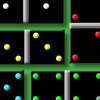Dominoes Environment

Age 5 to 11 Challenge Level:

These interactive dominoes can be dragged around the screen.Seven Flipped

Age 7 to 11 Challenge Level:

Investigate the smallest number of moves it takes to turn these mats upside-down if you can only turn exactly three at a time.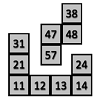100 Square Jigsaw

Age 5 to 7 Challenge Level:

Can you complete this jigsaw of the 100 square?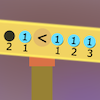Number Balance

Age 5 to 7 Challenge Level:

Can you hang weights in the right place to make the equaliser balance?Arrangements

Age 7 to 11 Challenge Level:

Is it possible to place 2 counters on the 3 by 3 grid so that there is an even number of counters in every row and every column? How about if you have 3 counters or 4 counters or....?Growing Garlic

Age 5 to 7 Challenge Level:

Ben and his mum are planting garlic. Use the interactivity to help you find out how many cloves of garlic they might have had.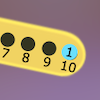Are You Well Balanced?

Age 5 to 7 Challenge Level:

Can you work out how to balance this equaliser? You can put more than one weight on a hook.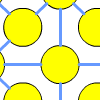One to Fifteen

Age 7 to 11 Challenge Level:

Can you put the numbers from 1 to 15 on the circles so that no consecutive numbers lie anywhere along a continuous straight line?Light the Lights

Age 5 to 7 Challenge Level:

Investigate which numbers make these lights come on. What is the smallest number you can find that lights up all the lights?Age 7 to 11 Challenge Level:

Three beads are threaded on a circular wire and are coloured either red or blue. Can you find all four different combinations?Junior Frogs

Age 5 to 11 Challenge Level:

Have a go at this well-known challenge. Can you swap the frogs and toads in as few slides and jumps as possible?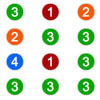Winning the Lottery

Age 7 to 11 Challenge Level:

Try out the lottery that is played in a far-away land. What is the chance of winning?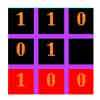Odd Tic Tac

Age 5 to 7 Challenge Level:

An odd version of tic tac toeMore Transformations on a Pegboard

Age 7 to 11 Challenge Level:

Use the interactivity to find all the different right-angled triangles you can make by just moving one corner of the starting triangle.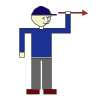Turning Man

Age 5 to 7 Challenge Level:

Use the interactivity to find out how many quarter turns the man must rotate through to look like each of the pictures.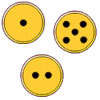Fair Exchange

Age 5 to 7 Challenge Level:

In your bank, you have three types of coins. The number of spots shows how much they are worth. Can you choose coins to exchange with the groups given to make the same total?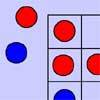Red Even

Age 7 to 11 Challenge Level:

You have 4 red and 5 blue counters. How many ways can they be placed on a 3 by 3 grid so that all the rows columns and diagonals have an even number of red counters?Part the Piles

Age 7 to 11 Challenge Level:

Try to stop your opponent from being able to split the piles of counters into unequal numbers. Can you find a strategy?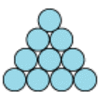Difference

Age 7 to 11 Challenge Level:

Place the numbers 1 to 10 in the circles so that each number is the difference between the two numbers just below it.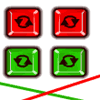Right Angle Challenge

Age 5 to 7 Challenge Level:

How many right angles can you make using two sticks?Coded Hundred Square

Age 7 to 11 Challenge Level:

This 100 square jigsaw is written in code. It starts with 1 and ends with 100. Can you build it up?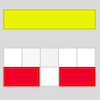Cuisenaire Counting

Age 5 to 7 Challenge Level:

Here are some rods that are different colours. How could I make a dark green rod using yellow and white rods?Coloured Squares

Age 5 to 7 Challenge Level:

Use the clues to colour each square.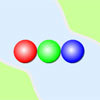Three Ball Line Up

Age 5 to 7 Challenge Level:

Use the interactivity to help get a feel for this problem and to find out all the possible ways the balls could land.Same Length Trains

Age 5 to 7 Challenge Level:

How many trains can you make which are the same length as Matt's, using rods that are identical?Making Trains

Age 5 to 7 Challenge Level:

Can you make a train the same length as Laura's but using three differently coloured rods? Is there only one way of doing it?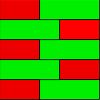Combining Cuisenaire

Age 7 to 11 Challenge Level:

Can you find all the different ways of lining up these Cuisenaire rods?Venn Diagrams

Age 5 to 11 Challenge Level:

Use the interactivities to complete these Venn diagrams.Multiplication Square Jigsaw

Age 7 to 11 Challenge Level:

Can you complete this jigsaw of the multiplication square?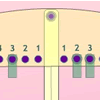Getting the Balance

Age 5 to 7 Challenge Level:

If you hang two weights on one side of this balance, in how many different ways can you hang three weights on the other side for it to be balanced?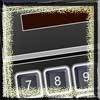Calculator Bingo

Age 7 to 11 Challenge Level:

A game to be played against the computer, or in groups. Pick a 7-digit number. A random digit is generated. What must you subract to remove the digit from your number? the first to zero wins.Multiples Grid

Age 7 to 11 Challenge Level:

What do the numbers shaded in blue on this hundred square have in common? What do you notice about the pink numbers? How about the shaded numbers in the other squares?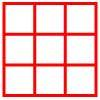Age 5 to 11 Challenge Level:

Place six toy ladybirds into the box so that there are two ladybirds in every column and every row.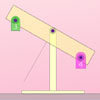Weighted Numbers

Age 5 to 7 Challenge Level:

Use the number weights to find different ways of balancing the equaliser.A Square of Numbers

Age 7 to 11 Challenge Level:

Can you put the numbers 1 to 8 into the circles so that the four calculations are correct?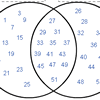Various Venns

Age 7 to 11 Challenge Level:

Use the interactivities to complete these Venn diagrams.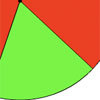Turning

Age 5 to 7 Challenge Level:

Use your mouse to move the red and green parts of this disc. Can you make images which show the turnings described?Fraction Card Game 1

Age 5 to 7 Challenge Level:

Match the halves.Odds or Sixes?

Age 7 to 11 Challenge Level:

Use the interactivity or play this dice game yourself. How could you make it fair?Two and One

Age 5 to 7 Challenge Level:

Terry and Ali are playing a game with three balls. Is it fair that Terry wins when the middle ball is red?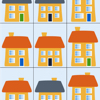Sort the Street

Age 5 to 7 Challenge Level:

Sort the houses in my street into different groups. Can you do it in any other ways?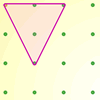Inside Triangles

Age 5 to 7 Challenge Level:

How many different triangles can you draw on the dotty grid which each have one dot in the middle?Matching Triangles

Age 5 to 7 Challenge Level:

Can you sort these triangles into three different families and explain how you did it?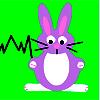Rabbit Run

Age 7 to 11 Challenge Level:

Ahmed has some wooden planks to use for three sides of a rabbit run against the shed. What quadrilaterals would he be able to make with the planks of different lengths?Fractions and Coins Game

Age 7 to 11 Challenge Level:

Work out the fractions to match the cards with the same amount of money.Tessellate the Triominoes

Age 5 to 7 Challenge Level:

What happens when you try and fit the triomino pieces into these two grids?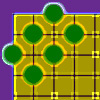Making Shapes

Age 5 to 7 Challenge Level:

Arrange any number of counters from these 18 on the grid to make a rectangle. What numbers of counters make rectangles? How many different rectangles can you make with each number of counters?One Million to Seven

Age 7 to 11 Challenge Level:

Start by putting one million (1 000 000) into the display of your calculator. Can you reduce this to 7 using just the 7 key and add, subtract, multiply, divide and equals as many times as you like?Triangle Animals

Age 5 to 7 Challenge Level:

How many different ways can you find to join three equilateral triangles together? Can you convince us that you have found them all?